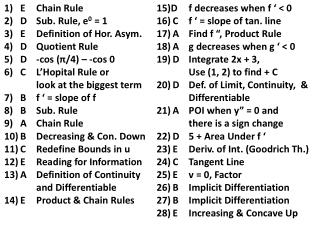DownloadDownload PresentationE Chain Rule D Sub. Rule, e 0 = 1 E Definition of Hor. Asym . D Quotient Rule

# E Chain Rule D Sub. Rule, e 0 = 1 E Definition of Hor. Asym . D Quotient Rule

Télécharger la présentation## E Chain Rule D Sub. Rule, e 0 = 1 E Definition of Hor. Asym . D Quotient Rule

- - - - - - - - - - - - - - - - - - - - - - - - - - - E N D - - - - - - - - - - - - - - - - - - - - - - - - - - -
##### Presentation Transcript

1. E Chain Rule D Sub. Rule, e0 = 1 E Definition of Hor. Asym. D Quotient Rule D -cos (π/4) – -cos 0 C L’Hopital Rule or look at the biggest term B f ‘ = slope of f B Sub. Rule A Chain Rule B Decreasing & Con. Down C Redefine Bounds in u E Reading for Information A Definition of Continuity and Differentiable E Product & Chain Rules 15)D f decreases when f ‘ < 0 C f ‘ = slope of tan. line A Find f “, Product Rule A g decreases when g ‘ < 0 D Integrate 2x + 3, Use (1, 2) to find + C D Def. of Limit, Continuity, & Differentiable A POI when y” = 0 and there is a sign change D 5 + Area Under f ‘ E Deriv. of Int. (Goodrich Th.) C Tangent Line E v = 0, Factor B Implicit Differentiation B Implicit Differentiation E Increasing & Concave Up